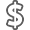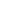# A Second Course in Statistics Regression Analysis

• ISBN13:

• ISBN10:

## 0321691695

• Edition: 7th
• Format: Hardcover
• Publisher: Pearson

Note: Supplemental materials are not guaranteed with Rental or Used book purchases.

Purchase Benefits

•Free Shipping On Orders Over \$35!
Your order must be \$35 or more to qualify for free economy shipping. Bulk sales, PO's, Marketplace items, eBooks and apparel do not qualify for this offer.
•Get Rewarded for Ordering Your Textbooks! Enroll Now
•We Buy This Book Back!
In-Store Credit: \$6.30
Check/Direct Deposit: \$6.00
PayPal: \$6.00
List Price: \$213.31 Save up to \$158.32
• Rent Book \$74.66Free Shipping

TERM
PRICE
DUE
IN STOCK USUALLY SHIPS WITHIN 24 HOURS.
*This item is part of an exclusive publisher rental program and requires an additional convenience fee. This fee will be reflected in the shopping cart.

### Supplemental Materials

What is included with this book?

• The New copy of this book will include any supplemental materials advertised. Please check the title of the book to determine if it should include any access cards, study guides, lab manuals, CDs, etc.
• The Used, Rental and eBook copies of this book are not guaranteed to include any supplemental materials. Typically, only the book itself is included. This is true even if the title states it includes any access cards, study guides, lab manuals, CDs, etc.

### Summary

A Second Course in Statistics: Regression Analysis, Seventh Edition, focuses on building linear statistical models and developing skills for implementing regression analysis in real situations. This text offers applications for engineering, sociology, psychology, science, and business. The authors use real data and scenarios extracted from news articles, journals, and actual consulting problems to show how to apply the concepts. In addition, seven case studies, now located throughout the text after applicable chapters, invite readers to focus on specific problems.

1. A Review of Basic Concepts (Optional)

1.1 Statistics and Data

1.2 Populations, Samples, and Random Sampling

1.3 Describing Qualitative Data

1.4 Describing Quantitative Data Graphically

1.5 Describing Quantitative Data Numerically

1.6 The Normal Probability Distribution

1.7 Sampling Distributions and the Central Limit Theorem

1.8 Estimating a Population Mean

1.9 Testing a Hypothesis About a Population Mean

1.10 Inferences About the Difference Between Two Population Means

1.11 Comparing Two Population Variances

2. Introduction to Regression Analysis

2.1 Modeling a Response

2.2 Overview of Regression Analysis

2.3 Regression Applications

2.4 Collecting the Data for Regression

3. Simple Linear Regression

3.1 Introduction

3.2 The Straight-Line Probabilistic Model

3.3 Fitting the Model: The Method of Least Squares

3.4 Model Assumptions

3.5 An Estimator of σ2

3.6 Assessing the Utility of the Model: Making Inferences About the Slope β1

3.7 The Coefficient of Correlation

3.8 The Coefficient of Determination

3.9 Using the Model for Estimation and Prediction

3.10 A Complete Example

3.11 Regression Through the Origin (Optional)

Case Study 1: Legal Advertising--Does It Pay?

4. Multiple Regression Models

4.1 General Form of a Multiple Regression Model

4.2 Model Assumptions

4.3 A First-Order Model with Quantitative Predictors

4.4 Fitting the Model: The Method of Least Squares

4.5 Estimation of σ2, the Variance of ε

4.6 Testing the Utility of a Model: The Analysis of Variance F-Test

4.7 Inferences About the Individual β Parameters

4.8 Multiple Coefficients of Determination: R2 and R2 adj

4.9 Using the Model for Estimation and Prediction

4.10 An Interaction Model with Quantitative Predictors

4.11 A Quadratic (Second-Order) Model with a Quantitative Predictor

4.12 More Complex Multiple Regression Models (Optional)

4.13 A Test for Comparing Nested Models

4.14 A Complete Example

Case Study 2: Modeling the Sale Prices of Residential Properties in Four Neighborhoods

5. Principles of Model Building

5.1 Introduction: Why Model Building is Important

5.2 The Two Types of Independent Variables: Quantitative and Qualitative

5.3 Models with a Single Quantitative Independent Variable

5.4 First-Order Models with Two or More Quantitative Independent Variables

5.5 Second-Order Models with Two or More Quantitative Independent Variables

5.6 Coding Quantitative Independent Variables (Optional)

5.7 Models with One Qualitative Independent Variable

5.8 Models with Two Qualitative Independent Variables

5.9 Models with Three or More Qualitative Independent Variables

5.10 Models with Both Quantitative and Qualitative Independent Variables

5.11 External Model Validation

6. Variable Screening Methods

6.1 Introduction: Why Use a Variable-Screening Method?

6.2 Stepwise Regression

6.3 All-Possible-Regressions Selection Procedure

6.4 Caveats

Case Study 3: Deregulation of the Intrastate Trucking Industry

7. Some Regression Pitfalls

7.1 Introduction

7.2 Observational Data Versus Designed Experiments

7.3 Parameter Estimability and Interpretation

7.4 Multicollinearity

7.5 Extrapolation: Predicting Outside the Experimental Region

7.6 Variable Transformations

8. Residual Analysis

8.1 Introduction

8.2 Plotting Residuals

8.3 Detecting Lack of Fit

8.4 Detecting Unequal Variances

8.5 Checking the Normality Assumption

8.6 Detecting Outliers and Identifying Influential Observations

8.7 Detection of Residual Correlation: The Durbin-Watson Test

Case Study 4: An Analysis of Rain Levels in California

Case Study 5: An Investigation of Factors Affecting the Sale Price of Condominium Units Sold at Public Auction

9. Special Topics in Regression (Optional)

9.1 Introduction

9.2 Piecewise Linear Regression

9.3 Inverse Prediction

9.4 Weighted Least Squares

9.5 Modeling Qualitative Dependent Variables

9.6 Logistic Regression

9.7 Ridge Regression

9.8 Robust Regression

9.9 Nonparametric Regression Models

10. Introduction to Time Series Modeling and Forecasting

10.1 What is a Time Series?

10.2 Time Series Components

10.3 Forecasting Using Smoothing Techniques (Optional)

10.4 Forecasting: The Regression Approach

10.5 Autocorrelation and Autoregressive Error Models

10.6 Other Models for Autocorrelated Errors (Optional)

10.7 Constructing Time Series Models

10.8 Fitting Time Series Models with Autoregressive Errors

10.9 Forecasting with Time Series Autoregressive Models

10.10 Seasonal Time Series Models: An Example

10.11 Forecasting Using Lagged Values of the Dependent Variable (Optional)

Case Study 6: Modeling Daily Peak Electricity Demands

11. Principles of Experimental Design

11.1 Introduction

11.2 Experimental Design Terminology

11.3 Controlling the Information in an Experiment

11.4 Noise-Reducing Designs

11.5 Volume-Increasing Designs

11.6 Selecting the Sample Size

11.7 The Importance of Randomization

12. The Analysis of Variance for Designed Experiments

12.1 Introduction

12.2 The Logic Behind an Analysis of Variance

12.3 One-Factor Completely Randomized Designs

12.4 Randomized Block Designs

12.5 Two-Factor Factorial Experiments

12.6 More Complex Factorial Designs (Optional)

12.7 Follow-Up Analysis: Tukey's Multiple Comparisons of Means

12.8 Other Multiple Comparisons Methods (Optional)

12.9 Checking ANOVA Assumptions

Case Study 7: Reluctance to Transmit Bad News: The MUM Effect

Appendix A: Derivation of the Least Squares Estimates of β0 and β1 in Simple Linear Regression

Appendix B: The Mechanics of a Multiple Regression Analysis

B.1 Introduction

B.2 Matrices and Matrix Multiplication

B.3 Identity Matrices and Matrix Inversion

B.4 Solving Systems of Simultaneous Linear Equations

B.5 The Least Squares Equations and Their Solution

B.6 Calculating SSE and s2

B.7 Standard Errors of Estimators, Test Statistics, and Confidence Intervals for β0, β1, ... , βk

B.8 A Confidence Interval for a Linear Function of the β Parameters; A Confidence Interval for E(y)

B.9 A Prediction Interval for Some Value of y to be Observed in the Future

Appendix C: A Procedure for Inverting a Matrix

Appendix D: Statistical Tables

Table D.1: Normal Curve Areas

Table D.2: Critical Values for Student's t

Table D.3: Critical Values for the F Statistic: F.10

Table D.4: Critical Values for the F Statistic: F.05

Table D.5: Critical Values for the F Statistic: F.025

Table D.6: Critical Values for the F Statistic: F.01

Table D.7: Random Numbers

Table D.8: Critical Values for the Durbin-Watson d Statistic (α =.05)

Table D.9: Critical Values for the Durbin-Watson d Statistic (α =.01)

Table D.10: Critical Values for the X2-Statistic

Table D.11: Percentage Points of the Studentized Range, q(p,v), Upper 5%

Table D.12: Percentage Points of the Studentized Range, q(p,v), Upper 1%

Appendix E: File Layouts for Case Study Data Sets

Answers to Selected Odd Numbered Exercises

Index

Technology Tutorials: SAS, SPSS, MINITAB, and R (on CD)